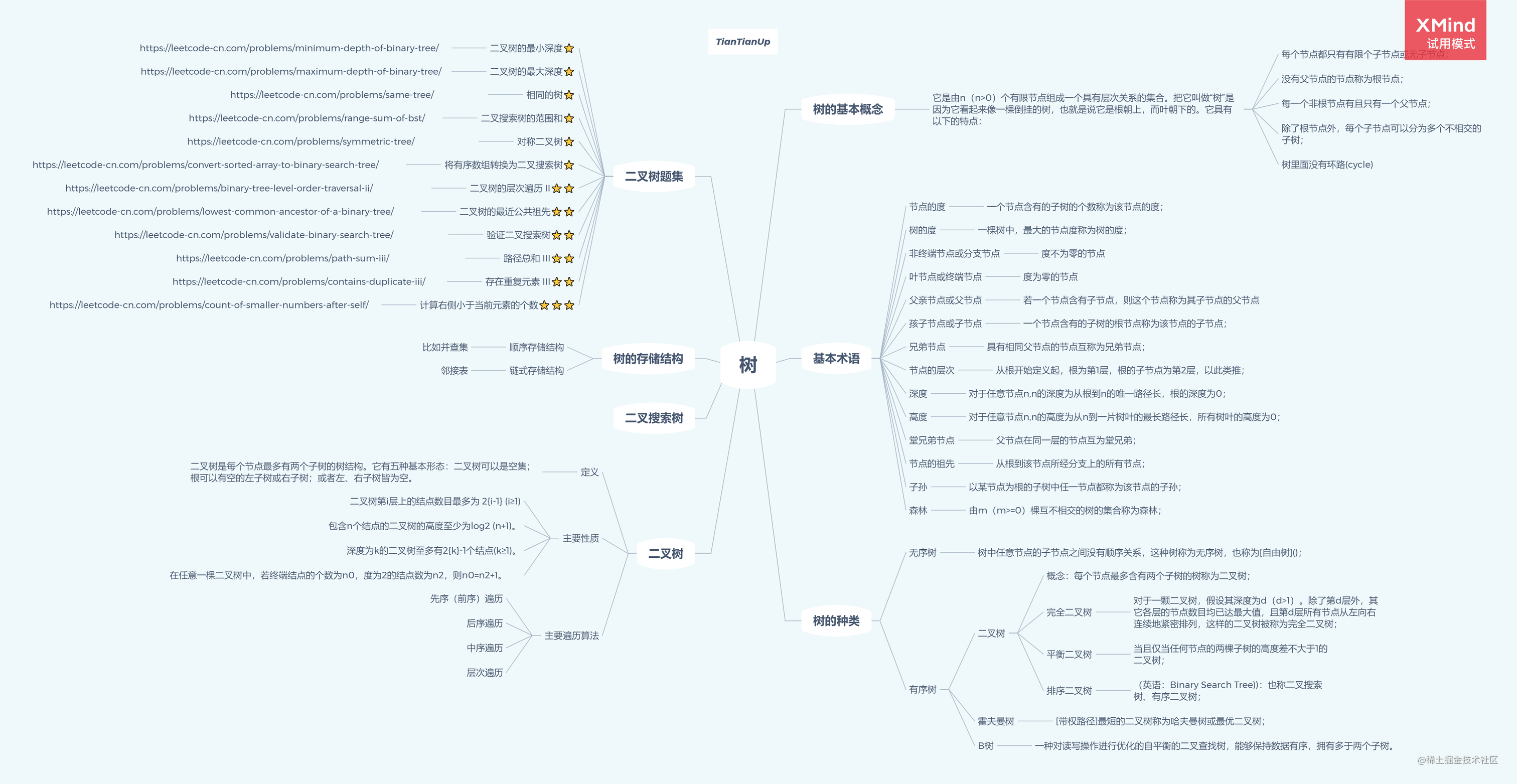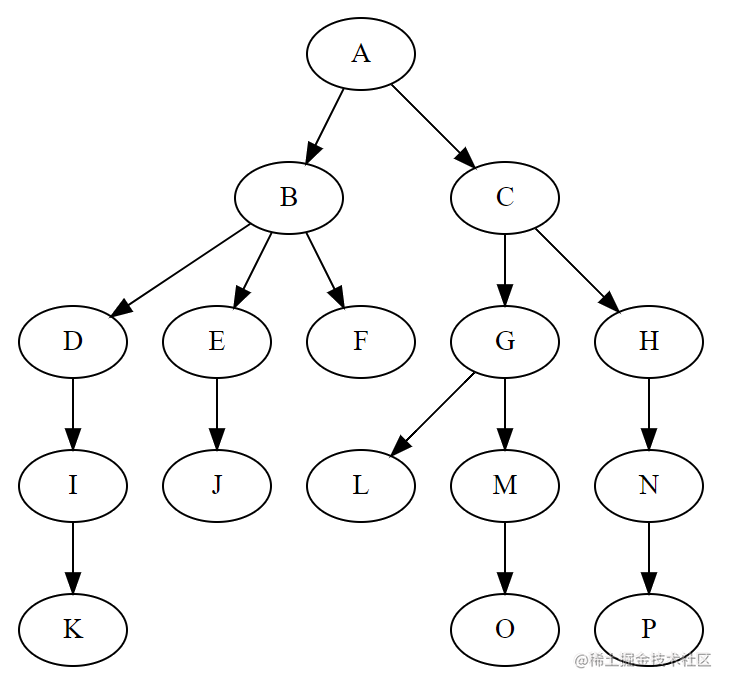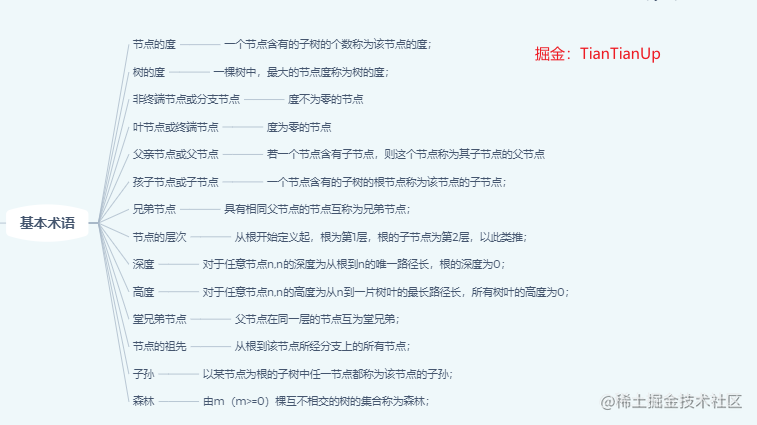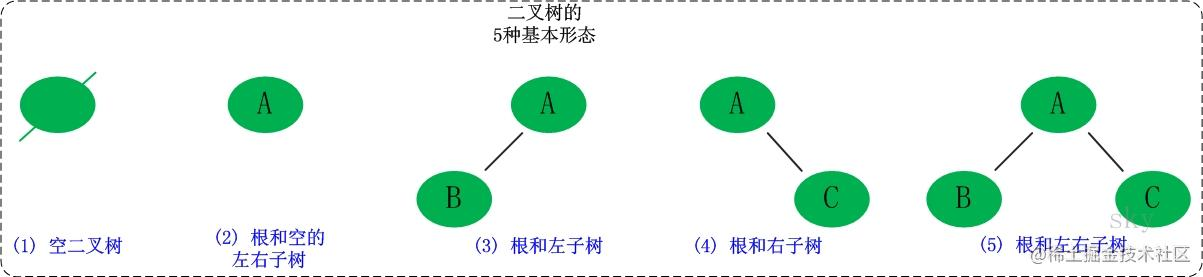# 「算法与数据结构」二叉树之美## 前言

• 树的基本概念
• 基本术语
• 树的种类
• 二叉树概念
• 二叉树的遍历
• 二叉树题目汇总## 树的基本概念

• 每个节点都只有有限个子节点或无子节点；
• 没有父节点的节点称为根节点；
• 每一个非根节点有且只有一个父节点；
• 除了根节点外，每个子节点可以分为多个不相交的子树；
• 树里面没有环路(cycle)• A节点作为根节点，没有父节点，所以是根节点。
• 除根节点（A）外，其他的节点都有父节点，并且每个节点只有有限个子节点或无子节点。
• 从某个节点开始，可以分为很多个子树，举个例子，从B节点开始，即是如此。

## 基本术语1. 节点的度：一个节点含有的子树的个数称为该节点的度；
2. 树的度：一棵树中，最大的节点度称为树的度；
3. 叶节点终端节点：度为零的节点；
4. 非终端节点分支节点：度不为零的节点；
5. 父亲节点父节点：若一个节点含有子节点，则这个节点称为其子节点的父节点；
6. 孩子节点子节点：一个节点含有的子树的根节点称为该节点的子节点；
7. 兄弟节点：具有相同父节点的节点互称为兄弟节点；
8. 节点的层次：从根开始定义起，根为第1层，根的子节点为第2层，以此类推；
9. 深度：对于任意节点n,n的深度为从根到n的唯一路径长，根的深度为0；
10. 高度：对于任意节点n,n的高度为从n到一片树叶的最长路径长，所有树叶的高度为0；
11. 堂兄弟节点：父节点在同一层的节点互为堂兄弟；
12. 节点的祖先：从根到该节点所经分支上的所有节点；
13. 子孙：以某节点为根的子树中任一节点都称为该节点的子孙；
14. 森林：由m（m>=0）棵互不相交的树的集合称为森林。

## 树的种类

• 无序树：树中任意节点的子节点之间没有顺序关系，这种树称为无序树，也称为自由树
• 有序树：树中任意节点的子节点之间有顺序关系，这种树称为有序树；
• 二叉树：每个节点最多含有两个子树的树称为二叉树；
• 完全二叉树：对于一颗二叉树，假设其深度为d（d>1）。除了第d层外，其它各层的节点数目均已达最大值，且第d层所有节点从左向右连续地紧密排列，这样的二叉树被称为完全二叉树；
• 满二叉树：所有叶节点都在最底层的完全二叉树；
• 平衡二叉树AVL树）：当且仅当任何节点的两棵子树的高度差不大于1的二叉树；
• 排序二叉树（英语：Binary Search Tree))：也称二叉搜索树、有序二叉树；
• 霍夫曼树带权路径最短的二叉树称为哈夫曼树或最优二叉树；
• B树：一种对读写操作进行优化的自平衡的二叉查找树，能够保持数据有序，拥有多于两个子树。

## 二叉树的概念## 二叉树的遍历

• 前序遍历
• 中序遍历
• 后序遍历

### 前序遍历

``````输入: [1,null,2,3]
1
\
2
/
3

``````//   TianTianUp
//   * function TreeNode(val, left, right) {
//   *     this.val = (val===undefined ? 0 : val)
//   *     this.left = (left===undefined ? null : left)
//   *     this.right = (right===undefined ? null : right)
//   * }
let preorderTraversal  = (root, arr = []) => {
if(root) {
arr.push(root.val)
preorderTraversal(root.left, arr)
preorderTraversal(root.right, arr)
}
return arr
}

• 根节点为目标节点，开始向它子节点遍历
• 1.访问目标节点
• 2.左孩子入栈 -> 直至左孩子为空的节点
• 3.节点出栈，以右孩子为目标节点，再依次执行1、2、3
``````  let preorderTraversal = (root, arr = []) => {
const stack = [], res = []
let current = root
while(current || stack.length > 0) {
while (current) {
res.push(current.val)
stack.push(current)
current = current.left
}
current = stack.pop()
current = current.right
}
return res
}

### 中序遍历

2 / 3

``````const inorderTraversal  = (root, arr = []) => {
if(root) {
inorderTraversal(root.left, arr)
arr.push(root.val)
inorderTraversal(root.right, arr)
}
return arr
}

``````const inorderTraversal = (root, arr = []) => {
const stack = [], res = []
let current = root
while(current || stack.length > 0) {
while (current) {
stack.push(current)
current = current.left
}
current = stack.pop()
res.push(current.val)
current = current.right
}
return res
}

### 后续遍历

1
2 / 3

``````const postorderTraversal  = (root, arr = []) => {
if(root) {
postorderTraversal(root.left, arr)
postorderTraversal(root.right, arr)
arr.push(root.val)
}
return arr
}

``````const postorderTraversal = (root, arr = []) => {
const stack = [], res = []
let current = root, last = null  // last指针记录上一个节点
while(current || stack.length > 0) {
while (current) {
stack.push(current)
current = current.left
}
current = stack[stack.length - 1]
if (!current.right || current.right == last) {
current = stack.pop()
res.push(current.val)
last = current
current = null              // 继续弹栈
} else {
current = current.right
}
}
return res
}

### 二叉树的层次遍历 ⭐⭐

3

/
9 20 /
15 7

[ , [9,20], [15,7] ]

``````const levelOrder = function(root) {
if(!root) return []
let res = []
dfs(root, 0, res)
return res
}

function dfs(root, step, res){
if(root){
if(!res[step]) res[step] = []
res[step].push(root.val)
dfs(root.left, step + 1, res)
dfs(root.right, step + 1, res)
}
}

``````const levelOrder = (root) => {
let queue = [], res = []
if (root) queue.push(root);
while (queue.length) {
let next_queue = [],
now_res = []
while (queue.length) {
root = queue.shift()
now_res.push(root.val)
root.left && next_queue.push(root.left)
root.right && next_queue.push(root.right)
}
queue = next_queue
res.push(now_res)
}
return res
}

## ❤️ 感谢大家

1. 点赞支持下吧，让更多的人也能看到这篇内容（收藏不点赞，都是耍流氓 -_-）

2. 关注公众号前端UpUp，联系作者👉 DayDay2021 ，我们一起学习一起进步。

3. 觉得不错的话，也可以阅读TianTian近期梳理的文章（感谢掘友的鼓励与支持🌹🌹🌹）：